Electrostatics MSQ

# Electrostatics MSQ

Test Description

## 10 Questions MCQ Test Topic wise Tests for IIT JAM Physics | Electrostatics MSQ

Electrostatics MSQ for IIT JAM 2023 is part of Topic wise Tests for IIT JAM Physics preparation. The Electrostatics MSQ questions and answers have been prepared according to the IIT JAM exam syllabus.The Electrostatics MSQ MCQs are made for IIT JAM 2023 Exam. Find important definitions, questions, notes, meanings, examples, exercises, MCQs and online tests for Electrostatics MSQ below.
Solutions of Electrostatics MSQ questions in English are available as part of our Topic wise Tests for IIT JAM Physics for IIT JAM & Electrostatics MSQ solutions in Hindi for Topic wise Tests for IIT JAM Physics course. Download more important topics, notes, lectures and mock test series for IIT JAM Exam by signing up for free. Attempt Electrostatics MSQ | 10 questions in 45 minutes | Mock test for IIT JAM preparation | Free important questions MCQ to study Topic wise Tests for IIT JAM Physics for IIT JAM Exam | Download free PDF with solutions
 1 Crore+ students have signed up on EduRev. Have you?
*Multiple options can be correct
Electrostatics MSQ - Question 1

### A wire having a uniform linear charge density is bent in the form of a ring of radius R. Point A as shown in the figure, is in the plane of the ring but not at the centre. Two elements of the ring of lengths a1 and a2 subtend very small same angle at the point A. They are at distances r1 and r2 from the point a respectively.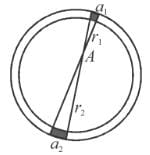Detailed Solution for Electrostatics MSQ - Question 1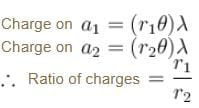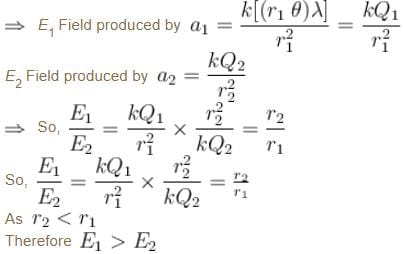i.e. Net field at A is towards a.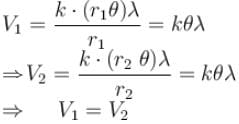The correct answers are: The ratio of charge of elements a1 and a2 is  r1/r2, The element a1 produced greater magnitude of electric field at A than element a2, The elements a1 and a2 produce same potential at A, The direction of net electric field at A is towards element  a2

*Multiple options can be correct
Electrostatics MSQ - Question 2

### In front of an earthed conductor a point charge +q is placed as shown in figure :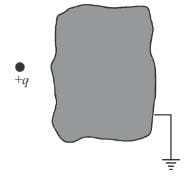Detailed Solution for Electrostatics MSQ - Question 2

Charge is distributed over the surface of conductor in such a way that net field due to this charge and outside charge q is zero inside. Field due to only q is non-zero.

The correct answers are: On the surface of conductor the net charge is negative., On the surface of conductor at some points charges are negative and at some points charges may be positive distributed non uniformly, Inside the conductor electric field due to point charge is non zero

*Multiple options can be correct
Electrostatics MSQ - Question 3

### Two concentric rings of radii R1 =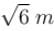and R2 = 4m are placed in yz plane with their centres at origin. They have uniform charge –q  and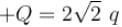on the inner and outer rings respectively.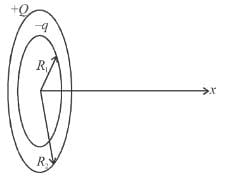Detailed Solution for Electrostatics MSQ - Question 3

V at origin ≠ 0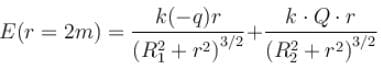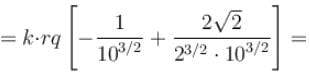0

From origin to r = 2,  field is towards origin.

The correct answers are: The electric field intensity is zero at r = 2m, A positive charged particle disturbed from origin along the x-axis will restore back to origin, Where potential is maximum on the x-axis, field intensity is zero

*Multiple options can be correct
Electrostatics MSQ - Question 4

The diagram shows part of an evacuated tube in which a stream of electrons from an electron gun passes between a pair of parallel large deflecting plates. The vertical displacement of the electron beam as it leaves the parallel plates is x. (Do not consider gravity and the electron enter the deflecting region parallel to the plates)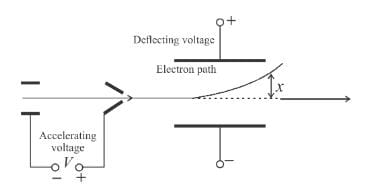Which one of A to D below will change the displacement x of the beam as it leaves the parallel plates

Detailed Solution for Electrostatics MSQ - Question 4

Increasing the accelerating voltage means increasing speed of the electron, thereby decreasing time spent between the plates. It will reduce x.
Increasing deflecting voltage means increasing electric field between the plates, making acceleration of electron greater. Increasing distance once again will change electric field between the plates.

The correct answers are: increasing the accelerating voltage, increasing the deflecting voltage, increasing the distance between the two deflecting plates

*Multiple options can be correct
Electrostatics MSQ - Question 5

In the figure shown, initially the spring of negligible mass is in undeformed state and the block has zero velocity E is a uniform electric field, then : (K = spring constant)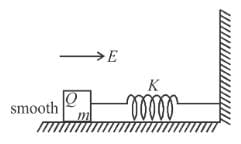Detailed Solution for Electrostatics MSQ - Question 5

Speed will be maximum when acceleration becomes zero. i.e. when Kx = EQ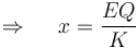By work-energy theorem :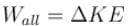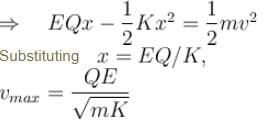The correct answers are: The maximum speed of the block will be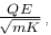The maximum compression of the spring will be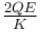*Multiple options can be correct
Electrostatics MSQ - Question 6

A particle of mass 2kg and charge 1mC is projected vertically with a velocity 10ms–1. There is a uniform horizontal electric field of 104N/C.

Detailed Solution for Electrostatics MSQ - Question 6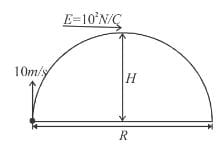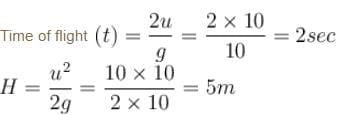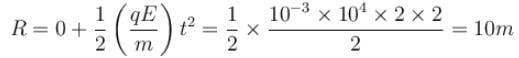The correct answers are: the horizontal range of the particle is 10m, the time of flight of the particle is 2sec, the maximum height reached is 5m

*Multiple options can be correct
Electrostatics MSQ - Question 7

An electric dipole is placed at the centre of a sphere. Mark the correct options.

Detailed Solution for Electrostatics MSQ - Question 7

The correct answers are: The flux of the electric field through the sphere is zero, The electric field is not zero at any where on the sphere

*Multiple options can be correct
Electrostatics MSQ - Question 8

The figure shows a charge q placed inside a cavity in an uncharged conductor. Now if external electric field is switched on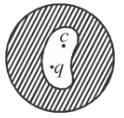Detailed Solution for Electrostatics MSQ - Question 8

The distribution of charge on the outer surface, depends only on the charges outside, and it distributes itself such that the net, electric field inside the outer surface due to the charge on outer surface and all the outer charges is zero. Similarly the distribution of charge on the inner surface, depends only on the charges inside the inner surface, and it distributes itself such that the net, electric field outside the inner surface due to the charge on inner surface and all the inner charges is zero.
Also the force on charge inside the cavity is due to the charge on the inner surface.

The correct answers are: only induced charge on inner surface will redistribute., both induced charge on outer and inner surface will redistribute., force on charge q placed inside the cavity will change.

*Multiple options can be correct
Electrostatics MSQ - Question 9

Mark the correct options

Detailed Solution for Electrostatics MSQ - Question 9

The correct answers are: The electric field calculated by Gauss’s law is the field due to all the charges, The flux of the electric field through a closed surface due to all the charges is equal to the flux due to the charges enclosed by the surface

*Multiple options can be correct
Electrostatics MSQ - Question 10

Two infinite, parallel, non-conducting sheets carry equal positive charge density σ. One is placed in the yz plane at x = 0 and the other at  x = a. Take potential V = 0 at  x = 0.

Detailed Solution for Electrostatics MSQ - Question 10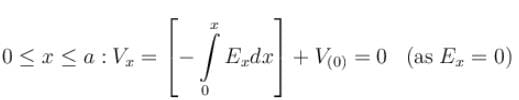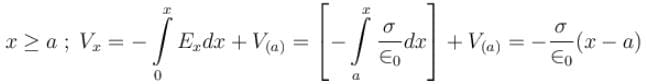; (As Va = 0)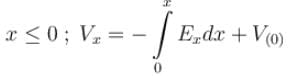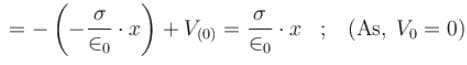The correct answers are: For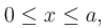potential Vx = 0, For x > a  potential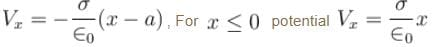## Topic wise Tests for IIT JAM Physics

217 tests
 Use Code STAYHOME200 and get INR 200 additional OFF Use Coupon Code